Updating search results...

# 12 Results

View
Selected filters:
• WI.Math.Content.HSG-GMD.A.1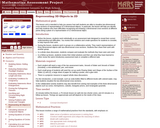Only Sharing Permitted
CC BY-NC-ND
Rating
0.0 stars

This lesson unit is intended to help teachers assess how well students are able to visualize two-dimensional cross-sections of representations of three-dimensional objects. In particular, the lesson will help you identify and help students who have difficulties recognizing and drawing two-dimensional cross-sections at different points along a plane of a representation of a three-dimensional object.

Subject:
Geometry
Mathematics
Material Type:
Assessment
Lesson Plan
Provider:
Shell Center for Mathematical Education
11/01/2017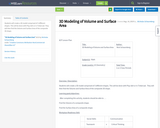Conditional Remix & Share Permitted
CC BY-NC-SA
Rating
0.0 stars

Students will create a 3D model comprised of 3 different shapes. This will be done with Play-doh or in Tinkercad. They will then find the Volume and Surface Area of the composite 3D shape.

Subject:
Education
Material Type:
Lesson
08/21/2019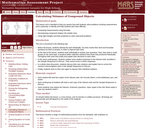Only Sharing Permitted
CC BY-NC-ND
Rating
0.0 stars

This lesson unit is intended to help teahcers assess how well students solve problems involving measurement, and in particular, to identify and help students who have the following difficulties; computing measurements using formulas; decomposing compound shapes into simpler ones; using right triangles and their properties to solve real-world problems.

Subject:
Geometry
Mathematics
Material Type:
Assessment
Lesson Plan
Provider:
Shell Center for Mathematical Education
11/01/2017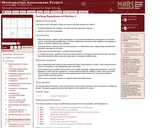Only Sharing Permitted
CC BY-NC-ND
Rating
0.0 stars

This lesson unit is intended to help teachers assess how well students are able to: translate between the equations of circles and their geometric features; and sketch a circle from its equation.

Subject:
Geometry
Mathematics
Material Type:
Assessment
Lesson Plan
Provider:
Shell Center for Mathematical Education
11/01/2017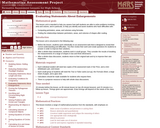Only Sharing Permitted
CC BY-NC-ND
Rating
0.0 stars

This lesson unit is intended to help teachers assess how well students are able to solve problems involving area and volume, and in particular, to help you identify and assist students who have difficulties with the following: computing perimeters, areas and volumes using formulas; and finding the relationships between perimeters, areas, and volumes of shapes after scaling.

Subject:
Geometry
Mathematics
Material Type:
Assessment
Lesson Plan
Provider:
Shell Center for Mathematical Education
11/01/2017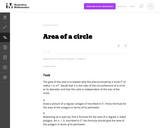Conditional Remix & Share Permitted
CC BY-NC-SA
Rating
0.0 stars

This is a task from the Illustrative Mathematics website that is one part of a complete illustration of the standard to which it is aligned. Each task has at least one solution and some commentary that addresses important asects of the task and its potential use. Here are the first few lines of the commentary for this task: The goal of this task is to explain why the area enclosed by a circle $C$ of radius $r$ is $\pi r^2$. Recall that $\pi$ is the ratio of the circumferen...

Subject:
Mathematics
Material Type:
Activity/Lab
Provider:
Illustrative Mathematics
Provider Set:
Illustrative Mathematics
Author:
Illustrative Mathematics
10/30/2013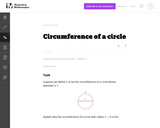Conditional Remix & Share Permitted
CC BY-NC-SA
Rating
0.0 stars

This is a task from the Illustrative Mathematics website that is one part of a complete illustration of the standard to which it is aligned. Each task has at least one solution and some commentary that addresses important asects of the task and its potential use. Here are the first few lines of the commentary for this task: Suppose we define $\pi$ to be the circumference of a circle whose diameter is 1: Explain why the circumference of a circle with radius $r \gt 0$ is \$2\...

Subject:
Mathematics
Material Type:
Activity/Lab
Provider:
Illustrative Mathematics
Provider Set:
Illustrative Mathematics
Author:
Illustrative Mathematics
10/30/2013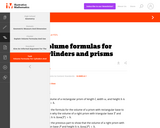Conditional Remix & Share Permitted
CC BY-NC-SA
Rating
0.0 stars

This is a task from the Illustrative Mathematics website that is one part of a complete illustration of the standard to which it is aligned. Each task has at least one solution and some commentary that addresses important aspects of the task and its potential use.

Subject:
Geometry
Mathematics
Material Type:
Activity/Lab
Provider:
Illustrative Mathematics
Provider Set:
Illustrative Mathematics
10/10/2017Conditional Remix & Share Permitted
CC BY-NC-SA
Rating
0.0 stars

This is a task from the Illustrative Mathematics website that is one part of a complete illustration of the standard to which it is aligned. Each task has at least one solution and some commentary that addresses important aspects of the task and its potential use.

Subject:
Geometry
Mathematics
Material Type:
Activity/Lab
Provider:
Illustrative Mathematics
Provider Set:
Illustrative Mathematics
10/10/2017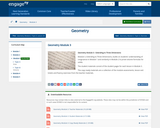Conditional Remix & Share Permitted
CC BY-NC-SA
Rating
0.0 stars

Module 3, Extending to Three Dimensions, builds on students understanding of congruence in Module 1 and similarity in Module 2 to prove volume formulas for solids. The student materials consist of the student pages for each lesson in Module 3. The copy ready materials are a collection of the module assessments, lesson exit tickets and fluency exercises from the teacher materials.

Subject:
Geometry
Mathematics
Material Type:
Module
Provider:
New York State Education Department
Provider Set:
EngageNY
07/03/2014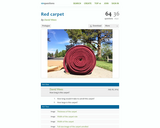Conditional Remix & Share Permitted
CC BY-NC-SA
Rating
0.0 stars

The activity begins with a photo of a large roll of red carpet.  What follows are the following questions:
1)  How long is the carpet?
2)  How long will it take to unroll the carpet?
3)  How large is the carpet?
What follows this first "ACT" are images and videos that provide students with more and more information that help them discover possible answers to these questions.

Subject:
Mathematics
Material Type:
Diagram/Illustration
Interactive
Lesson Plan
Simulation
Provider:
101 Questions
04/18/2016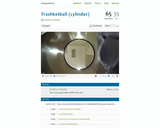Conditional Remix & Share Permitted
CC BY-NC
Rating
0.0 stars

This activity has the students calculate how many paperballs can fit into a trashcan.  There are many extensions that can be developed to use with this video.

Subject:
Mathematics
Material Type:
Lesson Plan
Provider:
101 Questions